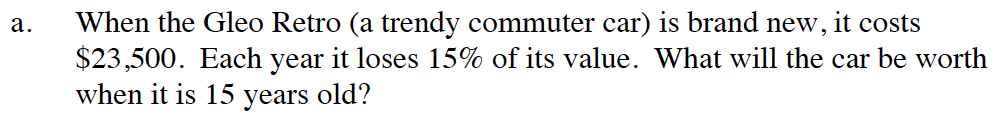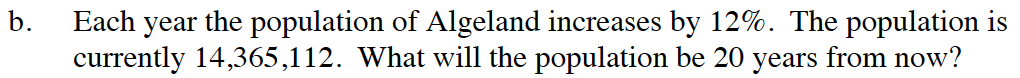Home > CC4 > Chapter 11 > Lesson 11.1.3 > Problem11-38

11-38.
1. Write equations to solve each of the following problems. Homework Help ✎

1. When the Gleo Retro (a trendy commuter car) is brand new, it costs $23,500. Each year it loses 15% of its value. What will the car be worth when it is 15 years old? 2. Each year the population of Algeland increases by 12%. The population is currently 14,365,112. What will the population be 20 years from now?y = 23500 · (0.85)x$2052.82y = 14,365,112 · (1.12)x